### Home > INT3 > Chapter 11 > Lesson 11.2.4 > Problem11-102

11-102.
1. Add, subtract, multiply, or divide the following rational expressions. Then, if possible, simplify your expression. Homework Help ✎

2.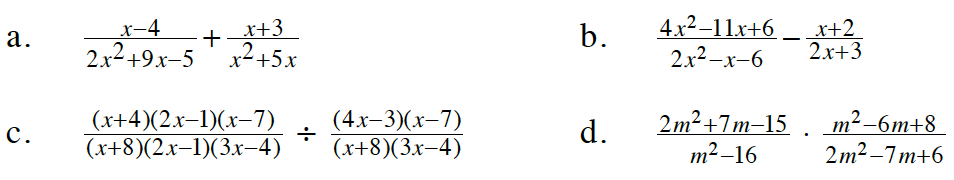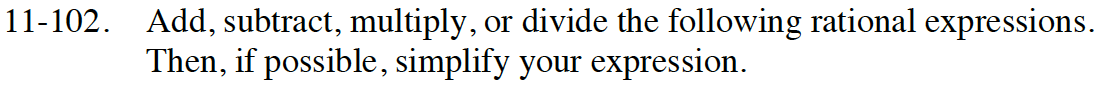See problems 11-42 and 11-57.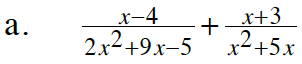In parts (a) and (b) you are adding and subtracting fractions. Therefore you will need a common denominator.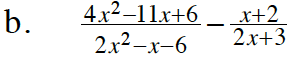$\frac{3x-5}{2x+3}$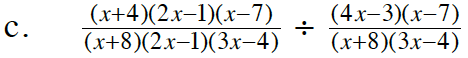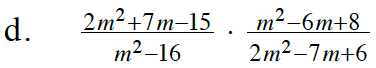Factor each expression.
Then remove factors that create Giant Ones.

$\frac{m+5}{m+4}$